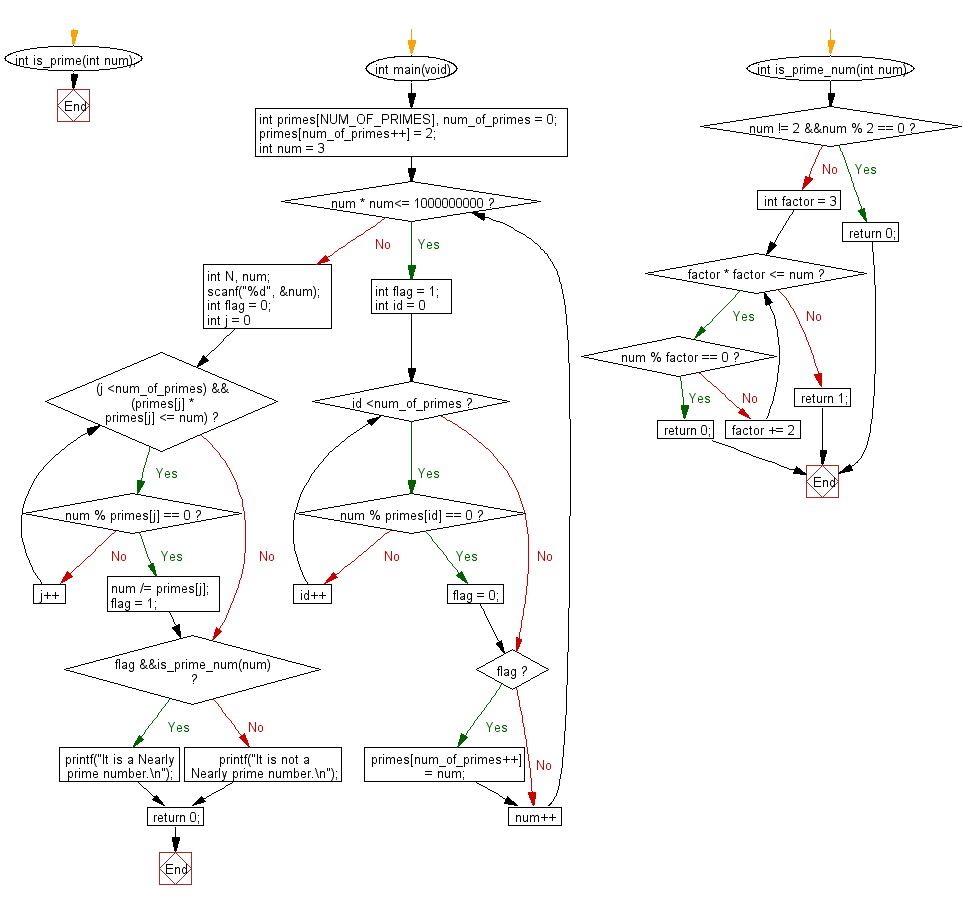﻿ C Program: Check if a given number is nearly prime or not - w3resource

# C Exercises: Check if a given number is nearly prime or not

## C Basic Declarations and Expressions: Exercise-93 with Solution

Write a C program to check if a given number is nearly prime number or not.
Nearly prime numbers are a positive integer which is equal to the product of two prime numbers

Sample Solution:

C Code:

``````#include <stdio.h>

// Define the number of primes to generate
#define NUM_OF_PRIMES 3500

// Function to check if a number is prime
int is_prime(int num);

int main() {
int primes[NUM_OF_PRIMES], num_of_primes = 0;

// Initialize the list of primes with the first prime number
primes[num_of_primes++] = 2;

// Generate primes up to 1000000000
for(int num = 3; num * num<= 1000000000; num++) {
int flag = 1;

// Check if num is divisible by any previously generated primes
for(int id = 0; id <num_of_primes; id++) {
if(num % primes[id] == 0) {
flag = 0;
break;
}
}

// If num is prime, add it to the list of primes
if(flag) primes[num_of_primes++] = num;
}

int N, num;
scanf("%d", &num);
int flag = 0;

// Check if num is a Nearly prime number
for(int j = 0; (j <num_of_primes) && (primes[j] * primes[j] <= num); j++) {
if(num % primes[j] == 0) {
num /= primes[j];
flag = 1;
break;
}
}

// Check if the remaining number after division is also prime
if(flag &&is_prime(num))
printf("It is a Nearly prime number.\n");
else
printf("It is not a Nearly prime number.\n");

return 0;
}

// Function to check if a number is prime
int is_prime(int num) {
if(num != 2 &&num % 2 == 0)
return 0;

for(int factor = 3; factor * factor <= num ; factor += 2) {
if(num % factor == 0)
return 0;
}

return 1;
}
``````

Sample Output:

```It is not a Nearly prime number.
```

Flowchart:C programming Code Editor:

What is the difficulty level of this exercise?

Test your Programming skills with w3resource's quiz.

﻿